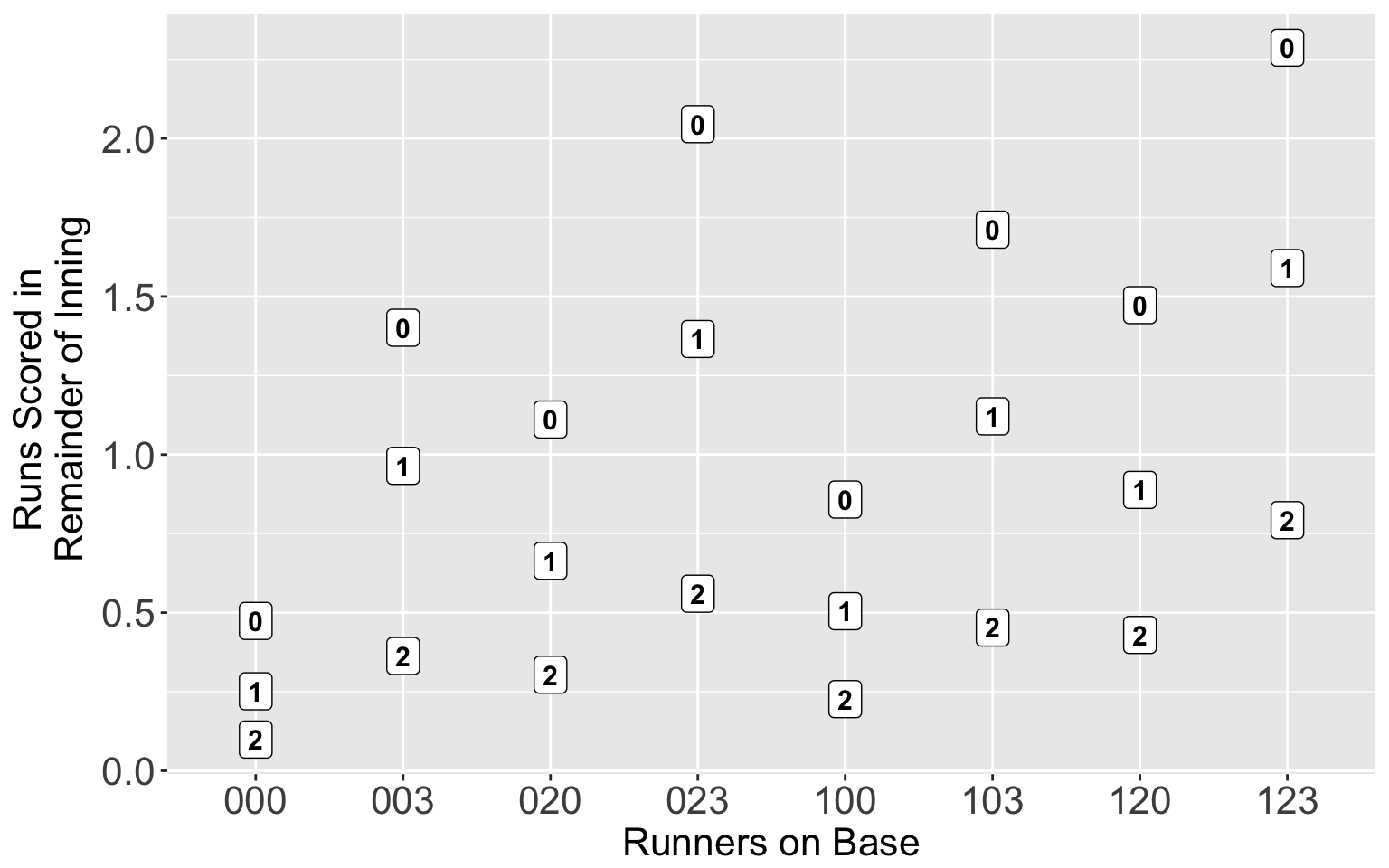### Chapter 3 - Runs Expectancy

This chapter illustrates graphing the famous runs expectancy matrix.

library(readr)
library(knitr)
library(ggplot2)

#### The Data

To obtain the runs expectancy matrix, one needs the Retrosheet play-by-play data for a particular season. I have computed the runs expectancies using 2015 season data. I have stored the data into a csv file that we read into R and store in the variable RR.

RR <- read_csv("https://bayesball.github.io/VB/data/runs2015.csv")

Use the kable function to display the data frame containing the runs expectancies.

kable(RR)
X1 STATE Mean Outs Bases O
1 000 0 0.4738828 OUTS = 0 000 0
2 000 1 0.2514400 OUTS = 1 000 1
3 000 2 0.0988068 OUTS = 2 000 2
4 001 0 1.4011407 OUTS = 0 003 0
5 001 1 0.9643617 OUTS = 1 003 1
6 001 2 0.3630464 OUTS = 2 003 2
7 010 0 1.1109418 OUTS = 0 020 0
8 010 1 0.6637977 OUTS = 1 020 1
9 010 2 0.3036562 OUTS = 2 020 2
10 011 0 2.0450000 OUTS = 0 023 0
11 011 1 1.3655761 OUTS = 1 023 1
12 011 2 0.5598688 OUTS = 2 023 2
13 100 0 0.8577522 OUTS = 0 100 0
14 100 1 0.5046115 OUTS = 1 100 1
15 100 2 0.2266157 OUTS = 2 100 2
16 101 0 1.7113951 OUTS = 0 103 0
17 101 1 1.1209412 OUTS = 1 103 1
18 101 2 0.4528302 OUTS = 2 103 2
19 110 0 1.4727344 OUTS = 0 120 0
20 110 1 0.8881782 OUTS = 1 120 1
21 110 2 0.4296086 OUTS = 2 120 2
22 111 0 2.2865412 OUTS = 0 123 0
23 111 1 1.5900901 OUTS = 1 123 1
24 111 2 0.7925729 OUTS = 2 123 2

### Graph of the Matrix

Here I am constructing a scatterplot of the Bases variable against the mean runs variable Mean where the plotting symbol is the O variable (number of outs).

ggplot(RR, aes(Bases, Mean, label=O)) +
geom_point(size=3) +
geom_label(color="black", size=4,
fontface="bold") +
ylab("Runs Scored in \n Remainder of Inning") +
xlab("Runners on Base") +
theme(axis.text = element_text(size=16),
axis.title = element_text(size=16))